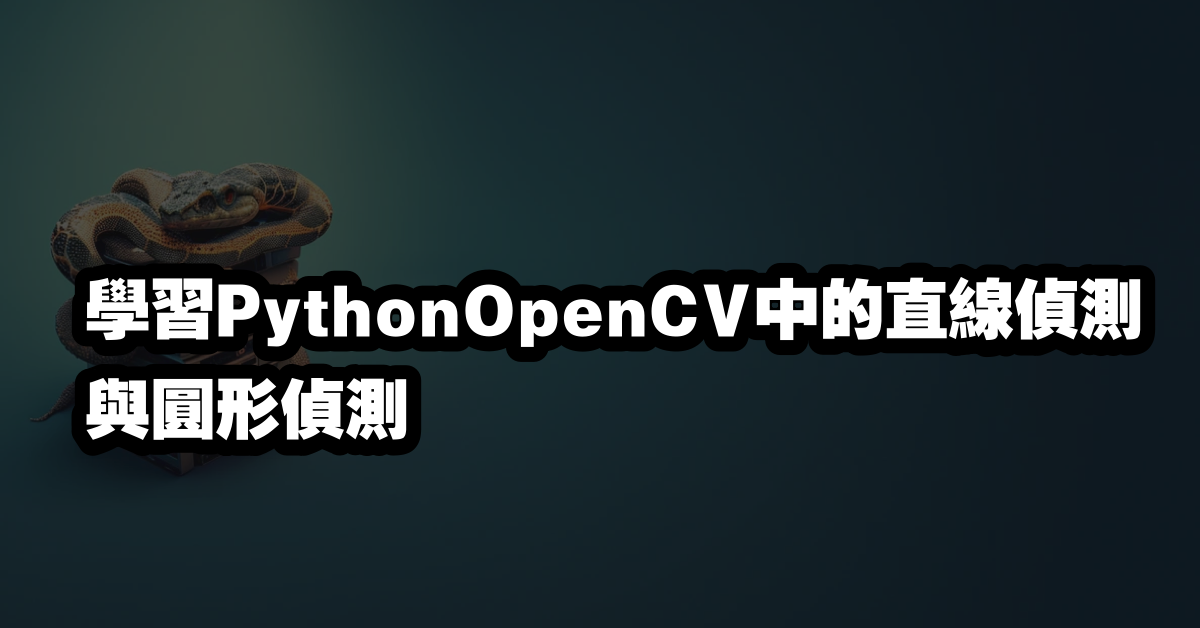# 學習PythonOpenCV中的直線偵測與圓形偵測# Python OpenCV 直線偵測與圓形偵測

Python OpenCV 是一個功能強大的影像處理套件，它可以讓開發者輕鬆地做出各種影像處理的應用，其中包括直線偵測與圓形偵測。本文將介紹如何使用 Python OpenCV 來做直線偵測與圓形偵測。

## Python OpenCV 直線偵測

Python OpenCV 直線偵測是一種影像處理技術，可以用來偵測圖像中的直線。它可以用來偵測圖像中的線條，例如邊緣、輪廓、輪廓等。

Python OpenCV 直線偵測的基本原理是：對圖像中的每個像素進行掃描，如果某個像素的像素值與其他像素的像素值有一定的差異，則該像素可能是一條直線的一部分。

Python OpenCV 直線偵測的基本步驟如下：

• 讀取圖像：使用 cv2.imread() 函數讀取圖像。
• 灰度轉換：使用 cv2.cvtColor() 函數將圖像轉換為灰度圖像。
• 邊緣偵測：使用 cv2.Canny() 函數對圖像進行邊緣偵測。
• 直線偵測：使用 cv2.HoughLines() 函數對圖像進行直線偵測。
• 繪製直線：使用 cv2.line() 函數將偵測到的直線繪製到圖像上。

import cv2

# 讀取圖像

# 灰度轉換
gray = cv2.cvtColor(img, cv2.COLOR_BGR2GRAY)

# 邊緣偵測
edges = cv2.Canny(gray, 50, 150, apertureSize = 3)

# 直線偵測
lines = cv2.HoughLines(edges, 1, np.pi/180, 200)

# 繪製直線
for line in lines:
rho, theta = line
a = np.cos(theta)
b = np.sin(theta)
x0 = a*rho
y0 = b*rho
x1 = int(x0 + 1000*(-b))
y1 = int(y0 + 1000*(a))
x2 = int(x0 - 1000*(-b))
y2 = int(y0 - 1000*(a))
cv2.line(img, (x1, y1), (x2, y2), (0, 0, 255), 2)

# 顯示圖像
cv2.imshow('image', img)
cv2.waitKey(0)
cv2.destroyAllWindows()


## Python OpenCV 圓形偵測

Python OpenCV 圓形偵測是一種影像處理技術，可以用來偵測圖像中的圓形。它可以用來偵測圖像中的圓形，例如圓形、橢圓形、椭圓形等。

Python OpenCV 圓形偵測的基本原理是：對圖像中的每個像素進行掃描，如果某個像素的像素值與其他像素的像素值有一定的差異，則該像素可能是一個圓形的一部分。

Python OpenCV 圓形偵測的基本步驟如下：

• 讀取圖像：使用 cv2.imread() 函數讀取圖像。
• 灰度轉換：使用 cv2.cvtColor() 函數將圖像轉換為灰度圖像。
• 邊緣偵測：使用 cv2.Canny() 函數對圖像進行邊緣偵測。
• 圓形偵測：使用 cv2.HoughCircles() 函數對圖像進行圓形偵測。
• 繪製圓形：使用 cv2.circle() 函數將偵測到的圓形繪製到圖像上。

import cv2

# 讀取圖像

# 灰度轉換
gray = cv2.cvtColor(img, cv2.COLOR_BGR2GRAY)

# 邊緣偵測
edges = cv2.Canny(gray, 50, 150, apertureSize = 3)

# 圓形偵測
circles = cv2.HoughCircles(edges, cv2.HOUGH_GRADIENT, 1, 20,

# 繪製圓形
for i in circles[0, :]:
cv2.circle(img, (i, i), i, (0, 255, 0), 2)

# 顯示圖像
cv2.imshow('image', img)
cv2.waitKey(0)
cv2.destroyAllWindows()